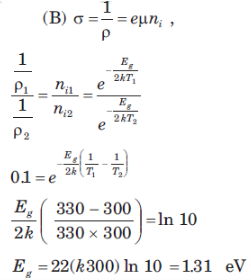Courses

# Test: Semiconductor Physics

## 10 Questions MCQ Test Electronic Devices | Test: Semiconductor Physics

Description
This mock test of Test: Semiconductor Physics for Electrical Engineering (EE) helps you for every Electrical Engineering (EE) entrance exam. This contains 10 Multiple Choice Questions for Electrical Engineering (EE) Test: Semiconductor Physics (mcq) to study with solutions a complete question bank. The solved questions answers in this Test: Semiconductor Physics quiz give you a good mix of easy questions and tough questions. Electrical Engineering (EE) students definitely take this Test: Semiconductor Physics exercise for a better result in the exam. You can find other Test: Semiconductor Physics extra questions, long questions & short questions for Electrical Engineering (EE) on EduRev as well by searching above.
QUESTION: 1

### In the problems assume the parameter given in following table. Use the temperature T= 300 K unless otherwise stated.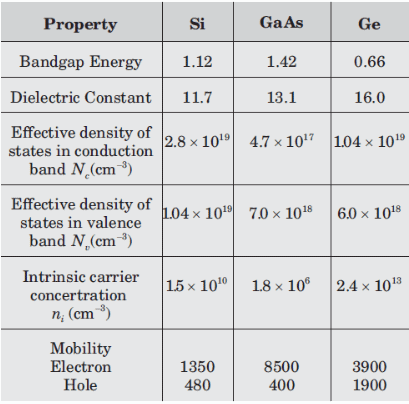In germanium semiconductor material at T 400 K the intrinsic concentration is (x 10^14 per cc)

Solution: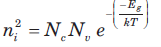QUESTION: 2

Solution:
QUESTION: 3

### Two semiconductor material have exactly the same properties except that material A has a bandgap of 1.0eV and material B has a bandgap energy of 1.2 eV. The ratio of intrinsic concentration of material A to that ofmaterial B is

Solution: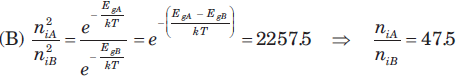QUESTION: 4

In silicon at T = 300 K the thermal-equilibrium concentration of electron is n0 = 5 x 10^4 cc. The hole concentration is

Solution:

ni x ni = no x po.

QUESTION: 5

In silicon at T = 300 K if the Fermi energy is 0.22 eV above the valence band energy, the value of p0 is​

Solution: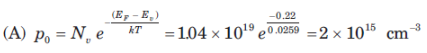QUESTION: 6

The thermal-equilibrium concentration of hole p0 in silicon at T = 300 K is 1015 cm3. The value of n0 is

Solution: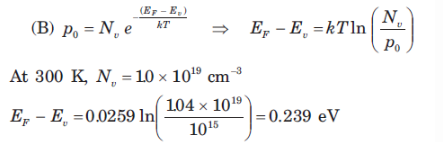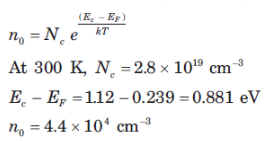QUESTION: 7

In germanium semiconductor at T 300 K, the acceptor concentrations is Na 1013 cm3 and donor concentration is Nd 0. The thermal equilibrium concentration p0 is

Solution: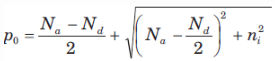QUESTION: 8

A silicon sample doped n type at 10^18 cm3 have a resistance of 10 ohm. The sample has an area of 10^(-6) cm2 and a length of 10 µm . The doping efficiency of the sample is (µn = 800 cm2/V-s )

Solution: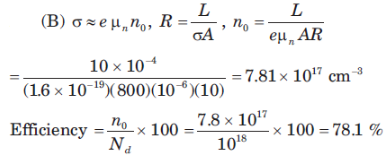QUESTION: 9

Six volts is applied across a 2 cm long semiconductor bar. The average drift velocity is 104 cms. The electron mobility is

Solution: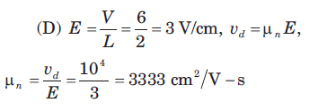QUESTION: 10

A particular intrinsic semiconductor has a resistivity of 50 (ohm-cm) at T = 300 K and 5 (ohm-cm) at T = 330 K. If change in mobility with temperature is neglected, the bandgap energy of the semiconductor is

Solution: